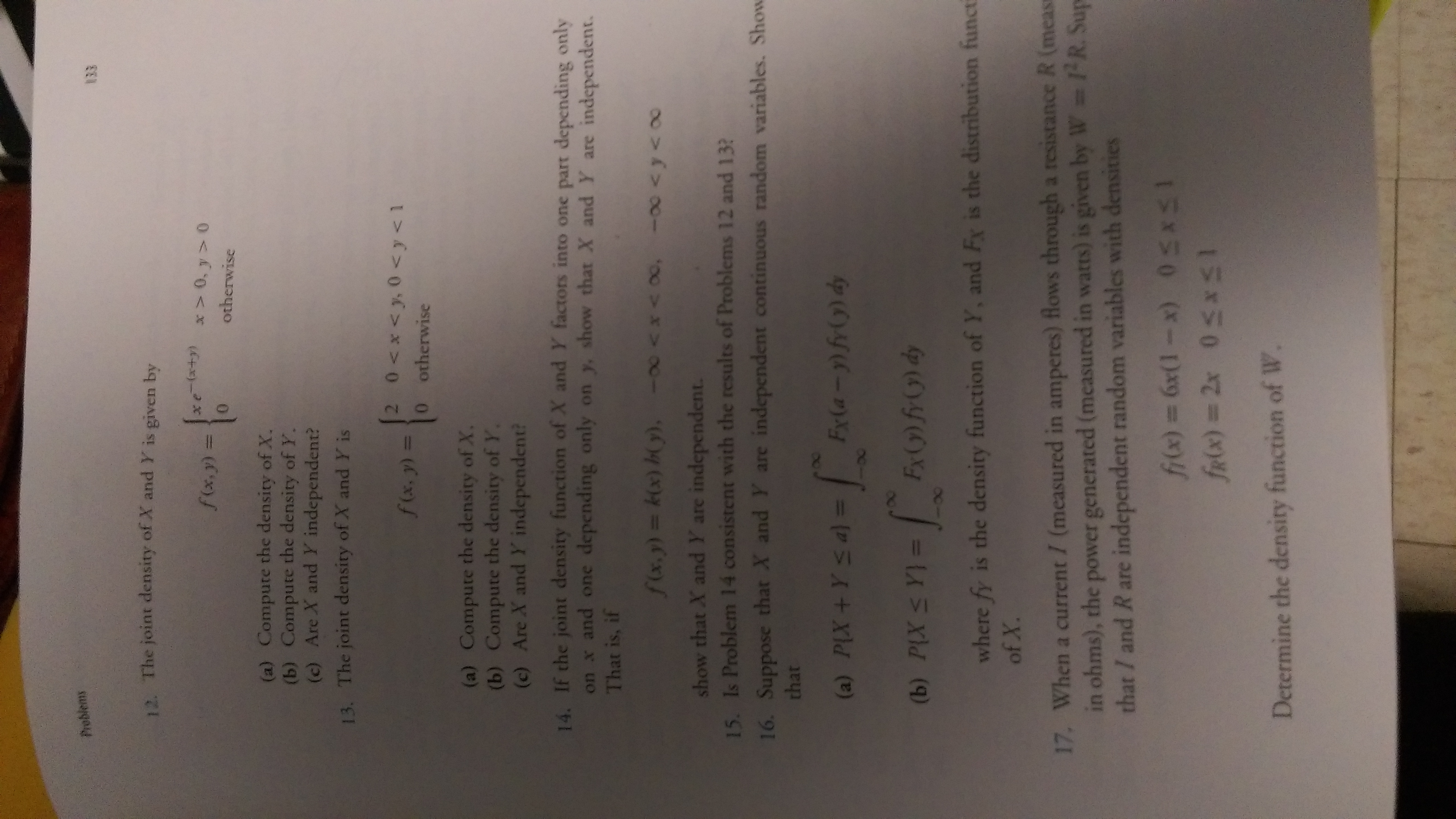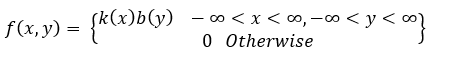# Problems12. The joint density of X and Y is given by(6+x)-*otherwisef(x,y) 3D(a) Compute the density of X.(b) Compute the density of Y.(c) Are X and Y independent?13. The joint density of X and Y isf(x,y) =0 otherwise(a) Compute the density of X.(b) Compute the density of Y.(c) Are X and Y independent?14. If the joint density function of X and Y factors into one part depending onlyon x and one depending only on y, show that X and Y are independent.That is, ifshow that X and Y are independent.15. Is Problem 14 consistent with the results of Problems 12 and 13?16. Suppose that X and Y are independent continuous random variables. Showthat(a) PIX+Ys a) =4Oy(->>-J(b) PLX S Y}p (6)YOL =where fy is the density function of Y, and Fx is the distribution functi17. When a current / (measured in amperes) flows through a resistance R (measin ohms), the power generated (measured in watts) is given by W R. Supthat / and R are independent random variables with densities15850 (X-1Da9 (x)4Ex50 %3D(x)YDetermine the density function of W.

Question
1 views

Q 14help_outlineImage TranscriptioncloseProblems 12. The joint density of X and Y is given by (6+x)-* otherwise f(x,y) 3D (a) Compute the density of X. (b) Compute the density of Y. (c) Are X and Y independent? 13. The joint density of X and Y is f(x,y) = 0 otherwise (a) Compute the density of X. (b) Compute the density of Y. (c) Are X and Y independent? 14. If the joint density function of X and Y factors into one part depending only on x and one depending only on y, show that X and Y are independent. That is, if show that X and Y are independent. 15. Is Problem 14 consistent with the results of Problems 12 and 13? 16. Suppose that X and Y are independent continuous random variables. Show that (a) PIX+Ys a) = 4Oy(->>-J (b) PLX S Y} p (6)YOL = where fy is the density function of Y, and Fx is the distribution functi 17. When a current / (measured in amperes) flows through a resistance R (meas in ohms), the power generated (measured in watts) is given by W R. Sup that / and R are independent random variables with densities 15850 (X-1Da9 (x)4 Ex50 %3D(x)Y Determine the density function of W. fullscreen
check_circle

Step 1

Given joint Density function is...

### Want to see the full answer?

See Solution

#### Want to see this answer and more?

Solutions are written by subject experts who are available 24/7. Questions are typically answered within 1 hour.*

See Solution
*Response times may vary by subject and question.
Tagged in